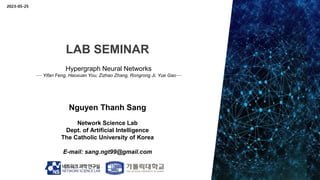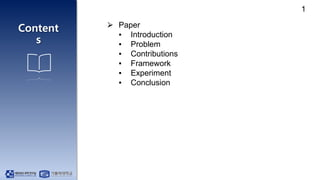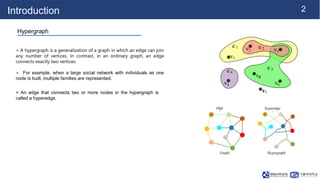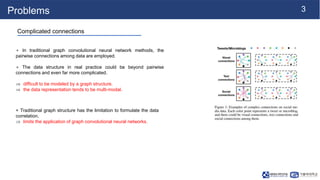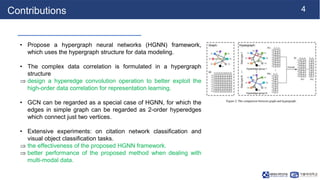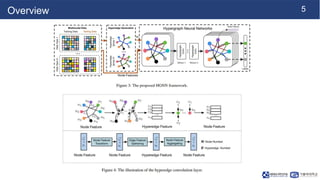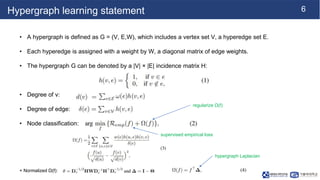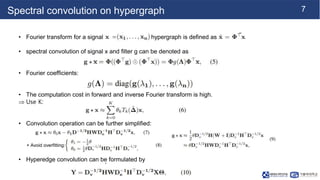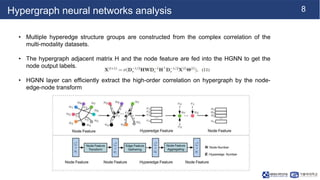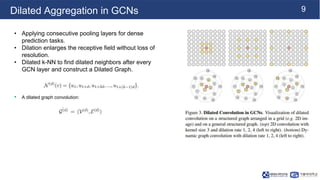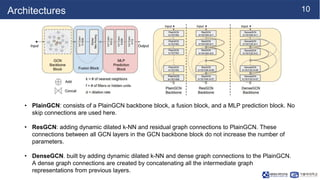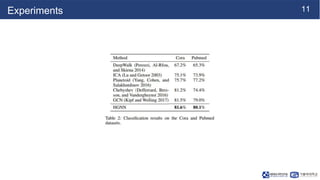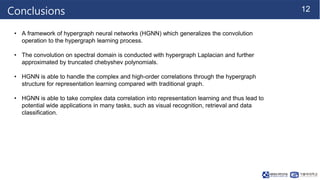1 of 14

### NS - CUK Seminar: S.T.Nguyen, Review on "Hypergraph Neural Networks", AAAI 2019

1. LAB SEMINAR Nguyen Thanh Sang Network Science Lab Dept. of Artificial Intelligence The Catholic University of Korea E-mail: sang.ngt99@gmail.com Hypergraph Neural Networks --- Yifan Feng, Haoxuan You, Zizhao Zhang, Rongrong Ji, Yue Gao--- 2023-05-25
2. Content s 1 ⮚ Paper ▪ Introduction ▪ Problem ▪ Contributions ▪ Framework ▪ Experiment ▪ Conclusion
3. 2 Introduction Hypergraph + A hypergraph is a generalization of a graph in which an edge can join any number of vertices. In contrast, in an ordinary graph, an edge connects exactly two vertices. + For example, when a large social network with individuals as one node is built, multiple families are represented. + An edge that connects two or more nodes in the hypergraph is called a hyperedge.
4. 3 Problems Complicated connections + In traditional graph convolutional neural network methods, the pairwise connections among data are employed. + The data structure in real practice could be beyond pairwise connections and even far more complicated.  difficult to be modeled by a graph structure.  the data representation tends to be multi-modal. + Traditional graph structure has the limitation to formulate the data correlation.  limits the application of graph convolutional neural networks.
5. 4 Contributions • Propose a hypergraph neural networks (HGNN) framework, which uses the hypergraph structure for data modeling. • The complex data correlation is formulated in a hypergraph structure  design a hyperedge convolution operation to better exploit the high-order data correlation for representation learning. • GCN can be regarded as a special case of HGNN, for which the edges in simple graph can be regarded as 2-order hyperedges which connect just two vertices. • Extensive experiments: on citation network classification and visual object classification tasks.  the effectiveness of the proposed HGNN framework.  better performance of the proposed method when dealing with multi-modal data.
6. 5 Overview
7. 6 Hypergraph learning statement • A hypergraph is defined as G = (V, E,W), which includes a vertex set V, a hyperedge set E. • Each hyperedge is assigned with a weight by W, a diagonal matrix of edge weights. • The hypergraph G can be denoted by a |V| × |E| incidence matrix H: • Degree of v: • Degree of edge: • Node classification: regularize Ω(f) hypergraph Laplacian supervised empirical loss + Normalized Ω(f):
8. 7 Spectral convolution on hypergraph • Fourier transform for a signal in hypergraph is defined as • spectral convolution of signal x and filter g can be denoted as • Fourier coefficients: • The computation cost in forward and inverse Fourier transform is high.  Use K: • Convolution operation can be further simplified: • Hyperedge convolution can be formulated by + Avoid overfitting:
9. 8 Hypergraph neural networks analysis • Multiple hyperedge structure groups are constructed from the complex correlation of the multi-modality datasets. • The hypergraph adjacent matrix H and the node feature are fed into the HGNN to get the node output labels. • HGNN layer can efficiently extract the high-order correlation on hypergraph by the node- edge-node transform
10. 9 Dilated Aggregation in GCNs • Applying consecutive pooling layers for dense prediction tasks. • Dilation enlarges the receptive field without loss of resolution. • Dilated k-NN to find dilated neighbors after every GCN layer and construct a Dilated Graph. • A dilated graph convolution:
11. 10 Architectures • PlainGCN: consists of a PlainGCN backbone block, a fusion block, and a MLP prediction block. No skip connections are used here. • ResGCN: adding dynamic dilated k-NN and residual graph connections to PlainGCN. These connections between all GCN layers in the GCN backbone block do not increase the number of parameters. • DenseGCN. built by adding dynamic dilated k-NN and dense graph connections to the PlainGCN. A dense graph connections are created by concatenating all the intermediate graph representations from previous layers.
12. 11 Experiments
13. 12 Conclusions • A framework of hypergraph neural networks (HGNN) which generalizes the convolution operation to the hypergraph learning process. • The convolution on spectral domain is conducted with hypergraph Laplacian and further approximated by truncated chebyshev polynomials. • HGNN is able to handle the complex and high-order correlations through the hypergraph structure for representation learning compared with traditional graph. • HGNN is able to take complex data correlation into representation learning and thus lead to potential wide applications in many tasks, such as visual recognition, retrieval and data classification.
14. 13 Thank you!